In this post a fuzzy approach to improve the diversity of population in genetic algorithm implementa- tions, based on Mamdani fuzzy rules, with the tuning of crossover and mutation probabilities, is proposed. The necessary steps to implement the adaptive genetic algorithm based on fuzzy rules is outlined, in which the crossover and mutation probabilities are changed based on a Mamdani fuzzy inference system, to improve the diversity of the population of the genetic algorithm. A numerical example in real codification of chromosomes shows the effectiveness and robustness of the adaptive technique in multimodals functions. This methodology is able to modify its properties in adaptive form, and can work with complex space search, resulting in a sufficiently robust method to optimize a variety of applications. It is demonstrated from computational results that the proposed methodology presents a better performance than an ordinary genetic algorithm.

The advantage of the proposed adaptive genetic algorithm is to allow finding global optimal solution of a multimodal function, maintaining the diversity of the population around constant value. In this post, so the genetic algorithm does not converge to local optimal solution, it is implemented a Mamdani fuzzy system based on linguistic variables which will describe the qualitative and quantitative performance, tuning the crossover and mutation probabilities. The proposed methodology consider the following steps:

# Step 1

The function to be optimized is defined. In this paper, the following multimodal function is chosen: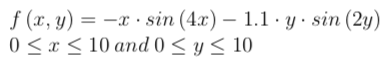Analyzing this function, the GA easily could converge to a local optimal solution if a good diversity of the population throughout the generations does not exist.

# Step 2

The number of generations, the size of the population, the number of variables, the initial crossoverprobability, and the initial mutation probability, are defined. A mechanism of evaluation of the chromosomes of the initial population is chosen.

# Step 3

The mechanism of selection of the GA is implemented. In this paper, is used the proportional selection by fitness, which was described in Section II. The crossover operator used is the linear crossover described in Section II, and Non-uniform mutation operator as well. For crossover and mutation operators it is necessary to consider the crossover and mutation probabilities Pc and Pm, respectively.

# Step 4

After the ordinary GA is designed, is necessary to analyze the diversity of the population. At this point is defined the fuzzy system, which will make decisions on Pc and Pm according to diversity of the population. The scheme of proposed genetic-fuzzy system.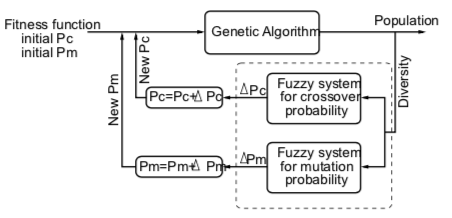the outputs of the fuzzy system to the crossover and mutation probabilities are ∆P . Therefore, after it is necessary to create a stage where these changes are adhered to the old probabil- ities so the new crossover and mutation probabilities can computed, and this fuzzy system makes the GA as adaptive, for different diversities of populations. The rule base of the fuzzy system is the following form: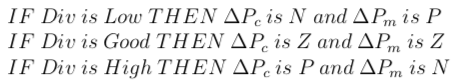where Div is the population diversity, N is the fuzzy set which represents −∆P , Z is the fuzzy set which represents the output near to zero, P is the fuzzy set which represents +∆P. With this rule base it has all knowledge base to make adaptive decisions in the genetic algorithm. In this implementation, it was used the Mamdani Min implication operator: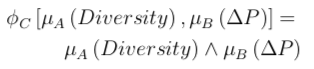It is necessary to unite all rules of the rule base in surfaces which will represent the knowledge of the fuzzy system, as follow: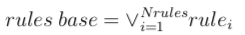and used to tune the crossover and mutation probabilities in the genetic algorithm. The fuzzy inference system was implemented by Max - Min Composition: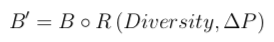# Step 5

Finally, the new crossover and mutation probabilities are obtained by:All basic concepts have been introduced so understand the Genetic-Fuzzy systems and facilitate the understanding the proposed methodology. The necessary steps to implement the adaptive genetic algorithm based on fuzzy rules was outlined, in which the crossover and mutation probabilities are changed based on a Mamdani fuzzy inference system, to improve the diversity of the population of the genetic algorithm. It has demonstrated from computational results that the proposed methodology presents a better performance than an ordinary genetic algorith. This is because it always adapt the genetic operators to find the global optimal solution without diminishing the diversity of the population. This is very important once the diversity be lost the algorithm can converge to local optimal solution. It was analyzed as vary the values of crossover and mutation probabilities, shown that these probabilities varied in a symmetrical form, and it is possible to change this property through the fuzzy system parameters. This methodology is able to modify its properties in adaptive form, and can work with complex multimodal functions, resulting in a sufficiently robust method to optimize a variety of applications.

*Source code : *